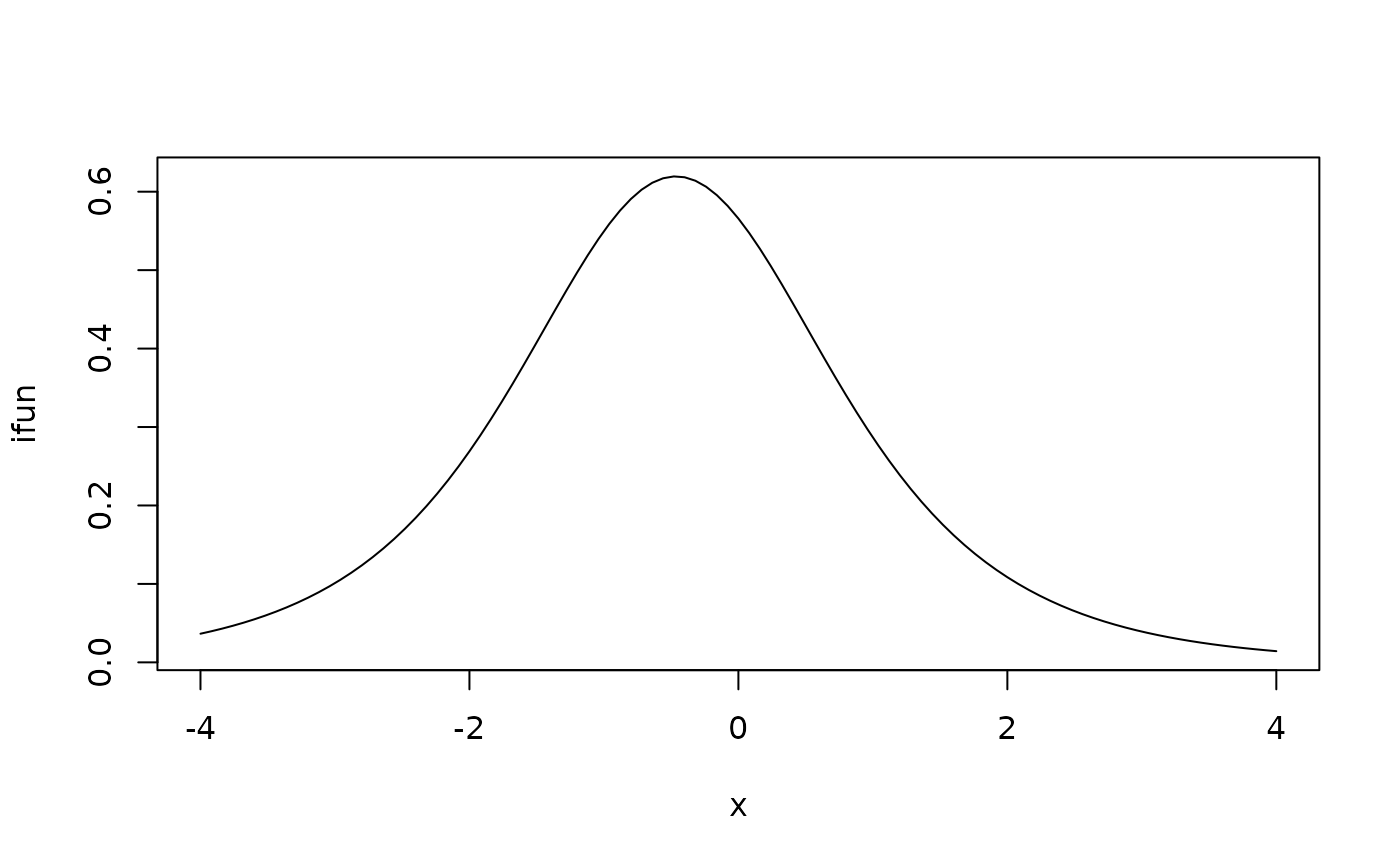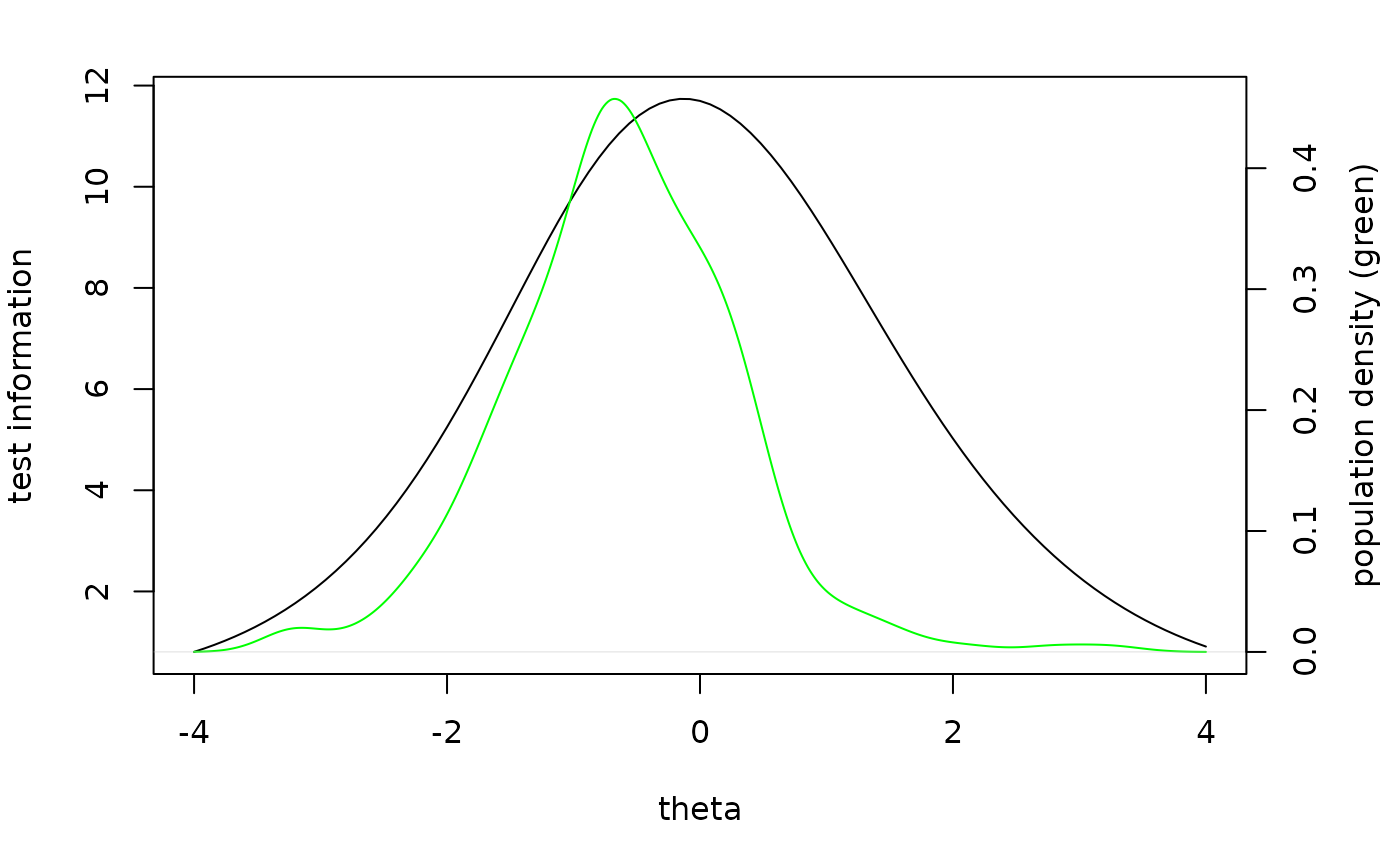returns information function, expected score function, score simulation function, or score distribution for a single item, an arbitrary group of items or all items

information(parms, items = NULL, booklet_id = NULL, which.draw = NULL)

expected_score(parms, items = NULL, booklet_id = NULL, which.draw = NULL)

r_score(parms, items = NULL, booklet_id = NULL, which.draw = NULL)

p_score(parms, items = NULL, booklet_id = NULL, which.draw = NULL)

## Arguments

parms

object produced by fit_enorm or a data.frame with columns item_id, item_score and, depending on parametrization, a column named either beta/delta, eta or b

items

vector of one or more item_id's. If NULL and booklet_id is also NULL, all items in parms are used

booklet_id

id of a single booklet (e.g. the test information function), if items is not NULL this is ignored

which.draw

the number of the random draw (only applicable if calibration method was Bayes). If NULL, the mean beta parameter will be used

## Value

Each function returns a new function which accepts a vector of theta's. These return the following values:

information

an equal length vector with the information estimate at each value of theta.

expected_score

an equal length vector with the expected score at each value of theta

r_score

a matrix with length(theta) rows and one column for each item containing simulated scores based on theta. To obtain test scores, use rowSums on this matrix

p_score

a matrix with length(theta) rows and one column for each possible sumscore containing the probability of the score given theta

## Examples


db = start_new_project(verbAggrRules,':memory:')
#> no column person_id provided, automatically generating unique person id's
#> $items #>  "S1DoCurse" "S1DoScold" "S1DoShout" "S1WantCurse" "S1WantScold" #>  "S1WantShout" "S2DoCurse" "S2DoScold" "S2DoShout" "S2WantCurse" #>  "S2WantScold" "S2WantShout" "S3DoCurse" "S3DoScold" "S3DoShout" #>  "S3WantCurse" "S3WantScold" "S3WantShout" "S4DoCurse" "S4DoScold" #>  "S4DoShout" "S4WantCurse" "S4WantScold" "S4WantShout" #> #>$person_properties
#> $columns_ignored #>  "gender" "anger" #> p = fit_enorm(db) # plot information function for single item ifun = information(p, "S1DoScold") plot(ifun,from=-4,to=4)# compare test information function to the population ability distribution ifun = information(p, booklet="agg") pv = plausible_values(db,p) op = par(no.readonly=TRUE) par(mar = c(5,4,2,4)) plot(ifun,from=-4,to=4, xlab='theta', ylab='test information') par(new=TRUE) plot(density(pv$PV1), col='green', axes=FALSE, xlab=NA, ylab=NA, main=NA)